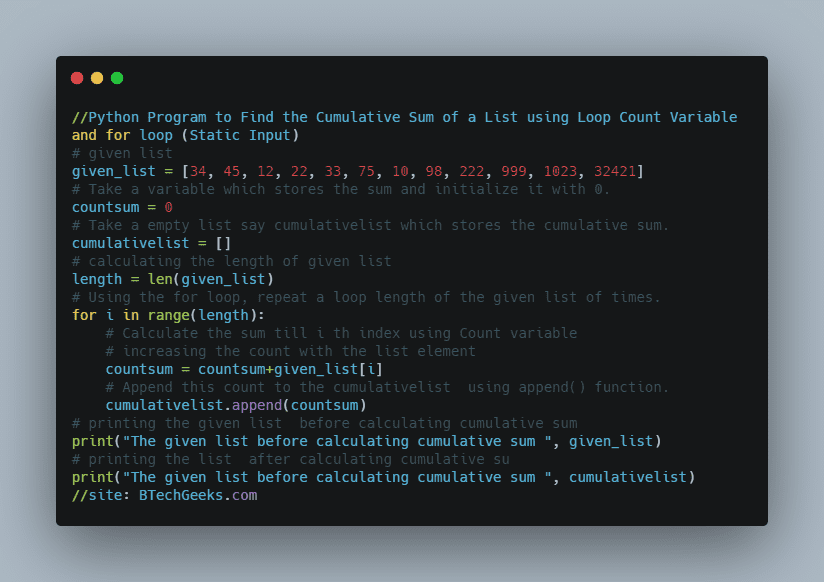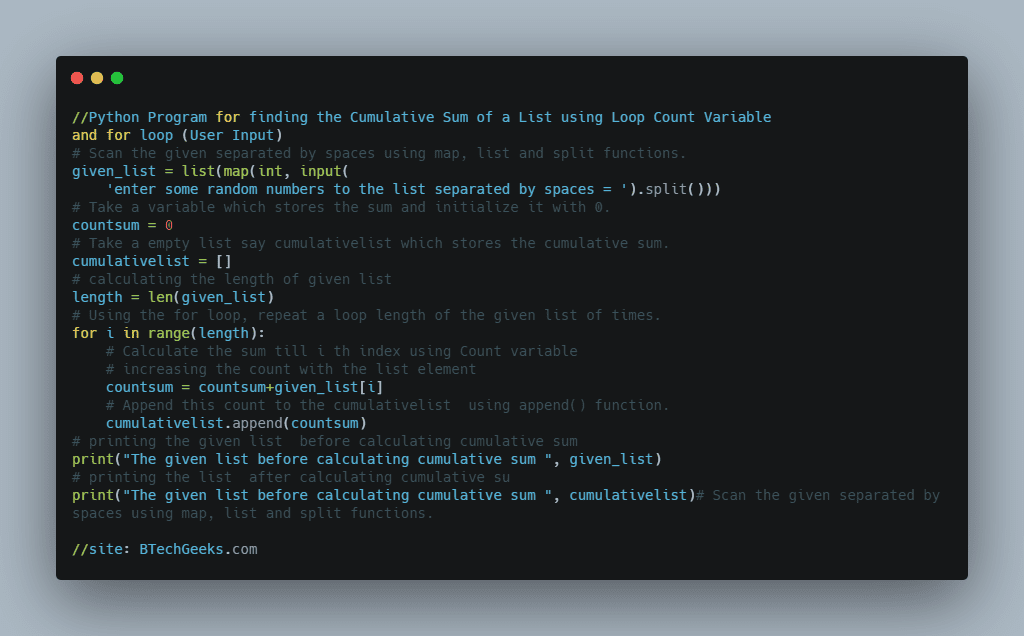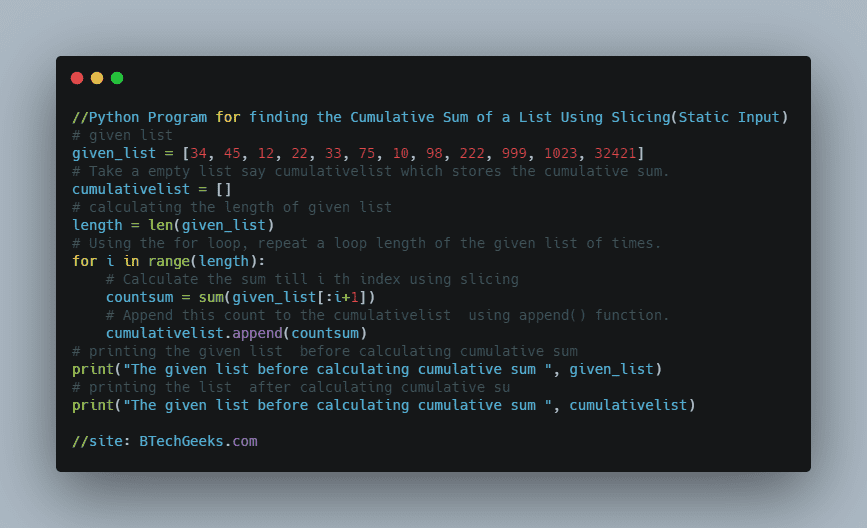# Python Program to Find the Cumulative Sum of a List using Different Methods | How to Calculate Cumulative Sum in Python with Examples?

The best and excellent way to learn a java programming language is by practicing Simple Java Program Examples as it includes basic to advanced levels of concepts.

Are you searching everywhere for a Program to Print the Cumulative Sum of Elements in a List? Then, this is the right place as it gives you a clear idea of what is meant by lists, the cumulative sum, different methods for finding the cumulative sum of numbers in a list, etc. Learn the simple codes for finding the cumulative sum of a list in python provided with enough examples and make the most out of them to write new codes on your own.

## Lists in Python

Python’s built-in container types are List and Tuple. Objects of both classes can store various additional objects that can be accessed via index. Lists and tuples, like strings, are sequence data types. Objects of different types can be stored in a list or a tuple.

A list is an ordered collection of objects (of the same or distinct types) separated by commas and surrounded by square brackets.

## Cumulative Sum

The cumulative total denotes “how much thus far” The cumulative sum is defined as the sum of a given sequence that grows or increases with successive additions. The growing amount of water in a swing pool is a real-world illustration of a cumulative accumulation.

Given a list, the task is to find the cumulative sum of the given list in python

### Cumulative Sum in Python Examples

Example 1:

Input:

given list = [34, 45, 12, 22, 33, 75, 10, 98, 222, 999, 1023, 32421]

Output:

The given list before calculating cumulative sum [34, 45, 12, 22, 33, 75, 10, 98, 222, 999, 1023, 32421]
The given list before calculating cumulative sum [34, 79, 91, 113, 146, 221, 231, 329, 551, 1550, 2573, 34994]

Example 2:

Input:

given list =[78, 45, 26, 95, 1, 2, 45, 13, 29, 39, 49, 68, 57, 13, 1, 2, 3, 1000, 2000, 100000]

Output:

The given list before calculating cumulative sum [78, 45, 26, 95, 1, 2, 45, 13, 29, 39, 49, 68, 57, 13, 1, 2, 3, 1000,
2000, 100000]
The given list before calculating cumulative sum [78, 123, 149, 244, 245, 247, 292, 305, 334, 373, 422, 490, 547,
560, 561, 563, 566, 1566, 3566, 103566]

## How to find the Cumulative Sum of Numbers in a List?

There are several ways to find the Cumulative sum in python some of them are:

Drive into Python Programming Examples and explore more instances related to python concepts so that you can become proficient in generating programs in Python Programming Language.

### Method #1:Using Count Variable and for loop (Static Input)

Approach:

• Give the list input as static
• Take a variable that stores the sum and initialize it with 0.
• Take an empty list say cumulative list which stores the cumulative sum.
• Using the for loop, repeat a loop length of the given list of times.
• Calculate the sum till i th index using Count variable.
• Append this count to the cumulative list using the append() function.
• Print the cumulative list.

### Write a Program to find the Cummulative Sum in a List?

# given list
given_list = [34, 45, 12, 22, 33, 75, 10, 98, 222, 999, 1023, 32421]
# Take a variable which stores the sum and initialize it with 0.
countsum = 0
# Take a empty list say cumulativelist which stores the cumulative sum.
cumulativelist = []
# calculating the length of given list
length = len(given_list)
# Using the for loop, repeat a loop length of the given list of times.
for i in range(length):
# Calculate the sum till i th index using Count variable
# increasing the count with the list element
countsum = countsum+given_list[i]
# Append this count to the cumulativelist  using append() function.
cumulativelist.append(countsum)
# printing the given list  before calculating cumulative sum
print("The given list before calculating cumulative sum ", given_list)
# printing the list  after calculating cumulative su
print("The given list before calculating cumulative sum ", cumulativelist)Output:

The given list before calculating cumulative sum  [34, 45, 12, 22, 33, 75, 10, 98, 222, 999, 1023, 32421]
The given list before calculating cumulative sum  [34, 79, 91, 113, 146, 221, 231, 329, 551, 1550, 2573, 34994]

### Method #2:Using Count Variable and for loop (User Input)

Approach:

• Scan the given separated by spaces using a map, list, and split functions.
• Take a variable that stores the sum and initialize it with 0.
• Take an empty list say cumulative list which stores the cumulative sum.
• Using the for loop, repeat a loop length of the given list of times.
• Calculate the sum till i th index using Count variable.
• Append this count to the cumulative list using the append() function.
• Print the cumulative list.

Below is the implementation:

# Scan the given separated by spaces using map, list and split functions.
given_list = list(map(int, input(
'enter some random numbers to the list separated by spaces = ').split()))
# Take a variable which stores the sum and initialize it with 0.
countsum = 0
# Take a empty list say cumulativelist which stores the cumulative sum.
cumulativelist = []
# calculating the length of given list
length = len(given_list)
# Using the for loop, repeat a loop length of the given list of times.
for i in range(length):
# Calculate the sum till i th index using Count variable
# increasing the count with the list element
countsum = countsum+given_list[i]
# Append this count to the cumulativelist  using append() function.
cumulativelist.append(countsum)
# printing the given list  before calculating cumulative sum
print("The given list before calculating cumulative sum ", given_list)
# printing the list  after calculating cumulative su
print("The given list before calculating cumulative sum ", cumulativelist)Output:

enter some random numbers to the list separated by spaces = 78 45 26 95 1 2 45 13 29 39 49 68 57 13 1 2 3 1000 2000 100000
The given list before calculating cumulative sum [78, 45, 26, 95, 1, 2, 45, 13, 29, 39, 49, 68, 57, 13, 1, 2, 3, 1000,
2000, 100000]
The given list before calculating cumulative sum [78, 123, 149, 244, 245, 247, 292, 305, 334, 373, 422, 490, 547,
560, 561, 563, 566, 1566, 3566, 103566]

### Method #3:Using Slicing (Static Input)

Approach:

• Give the list input as static
• Take an empty list say cumulative list which stores the cumulative sum.
• Using the for loop, repeat a loop length of the given list of times.
• Calculate the sum till ith index using slicing and sum function.
• Append this count to the cumulative list using the append() function.
• Print the cumulative list.

Below is the implementation:

# given list
given_list = [34, 45, 12, 22, 33, 75, 10, 98, 222, 999, 1023, 32421]
# Take a empty list say cumulativelist which stores the cumulative sum.
cumulativelist = []
# calculating the length of given list
length = len(given_list)
# Using the for loop, repeat a loop length of the given list of times.
for i in range(length):
# Calculate the sum till i th index using slicing
countsum = sum(given_list[:i+1])
# Append this count to the cumulativelist  using append() function.
cumulativelist.append(countsum)
# printing the given list  before calculating cumulative sum
print("The given list before calculating cumulative sum ", given_list)
# printing the list  after calculating cumulative su
print("The given list before calculating cumulative sum ", cumulativelist)Output:

The given list before calculating cumulative sum  [34, 45, 12, 22, 33, 75, 10, 98, 222, 999, 1023, 32421]
The given list before calculating cumulative sum  [34, 79, 91, 113, 146, 221, 231, 329, 551, 1550, 2573, 34994]